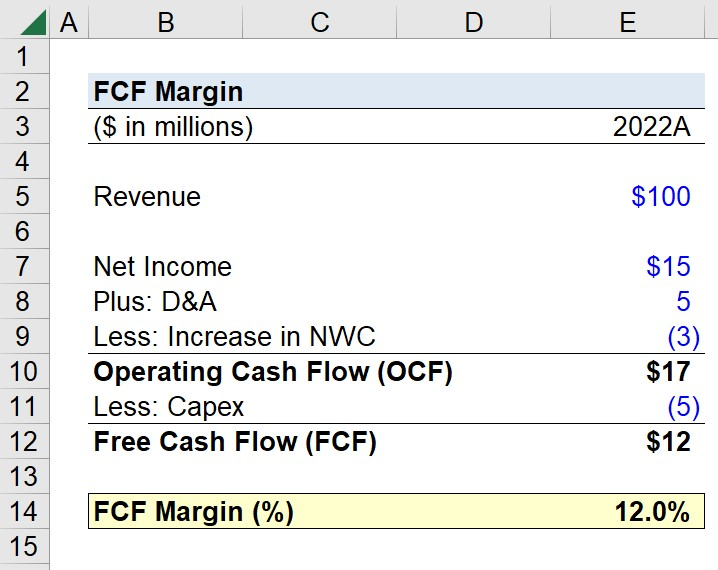Welcome to Wall Street Prep! Use code at checkout for 15% off.# FCF Margin

Guide to Understanding FCF Margin## How to Calculate Free Cash Flow Margin?

The FCF margin is a profitability ratio that compares a company’s free cash flow to its revenue to understand the proportion of revenue that becomes free cash flow (FCF).

The simplest variation of the FCF margin is calculated by taking a company’s cash flow from operations and deducting capital expenditures (Capex) since it is a recurring, core expense.

Free Cash Flow (FCF) = Operating Cash Flow – Capital Expenditure

There are several types of free cash flow metrics, such as free cash flow to firm (FCFF) and free cash flow to equity (FCFE), which are intended to measure a company’s discretionary cash flow. The difference between the metrics, however, comes down to the investor group(s) represented.

• Free Cash Flow to Firm (FCFF): FCFF is the cash generated by a company’s core operations that belongs to all capital providers, such as debt lenders and equity investors.
• Free Cash Flow to Equity (FCFE): FCFE refers to the residual cash for equity holders after the company’s operating costs (e.g. cost of goods sold and operating expenses like SG&A), re-investment needs, and mandatory financing-related outflows are deducted.

Each variation of free cash flow discussed thus far can be divided by revenue to reflect the proportion of a company’s revenue remaining for the investor group in the form of discretionary cash.

## What is a Good FCF Margin?

The FCF margin answers, “For each dollar of revenue generated, what percent gets converted into free cash flow (FCF)?”

Companies with an abundance of free cash flow available tend to operate more efficiently with higher profit margins, while possessing more cash to reinvest in their operations (i.e. funding working capital and capital expenditures).

Thus, the FCF margin portrays the efficiency at which a company can convert its revenue into free cash flow.

Furthermore, the FCF margin can also be useful for understanding a company’s capital intensity, i.e. the company’s reliance on capital expenditures to drive revenue and the percentage contribution from growth versus maintenance Capex.

## FCF Margin Formula

The FCF margin formula is as follows.

FCF Margin = (Operating Cash Flow Capital Expenditures) ÷ Revenue

The free cash flow metric we use here is the simplest variation, wherein a company’s capital expenditures are subtracted from its operating cash flow (OCF).

The starting point of the operating cash flow (OCF) calculation is net income from the accrual-based income statement.

From there, net income is adjusted for non-cash expenses (e.g. depreciation and amortization) and changes in net working capital (NWC).

Operating Cash Flow (OCF) = Net Income + Depreciation and Amortization Change in NWC

## FCF Margin Calculator

We’ll now move on to a modeling exercise, which you can access by filling out the form below.Submitting...

## FCF Margin Calculation Example

Suppose you’re tasked with calculating the FCF margin of a company that generated \$100 million in fiscal year 2022.

Over the same period, the company’s net income was \$15 million, D&A and Capex were both \$5 million, and the change in net working capital (NWC) was an increase of \$3 million.

• Net Income = \$15 million
• D&A = \$5 million
• Change in NWC = (\$3 million)
• Capex = (\$5 million)

To calculate the operating cash flow (OCF), we’ll add back the non-cash depreciation expense to net income and subtract the increase in NWC to arrive at an OCF of \$17 million.

• Operating Cash Flow (OCF) = \$15 million + \$5 million – \$3 million = \$17 million

The next step is to deduct our Capex assumption from our operating cash flow (OCF), which results in our company’s free cash flow (FCF).

• Free Cash Flow (FCF) = \$17 million – \$5 million = \$12 million

In 2022, our company’s free cash flow (FCF) was \$12 million, which we’ll divide by the net revenue generated in the corresponding period (\$100 million) to arrive at a FCF margin of 12%.

• FCF Margin (%) = \$12 million ÷ \$100 million = 12.0%### The Wharton Online and Wall Street Prep Private Equity Certificate Program

Level up your career with the world's most recognized private equity investing program. Enrollment is open for the Jan. 29 - Mar. 25 cohort.